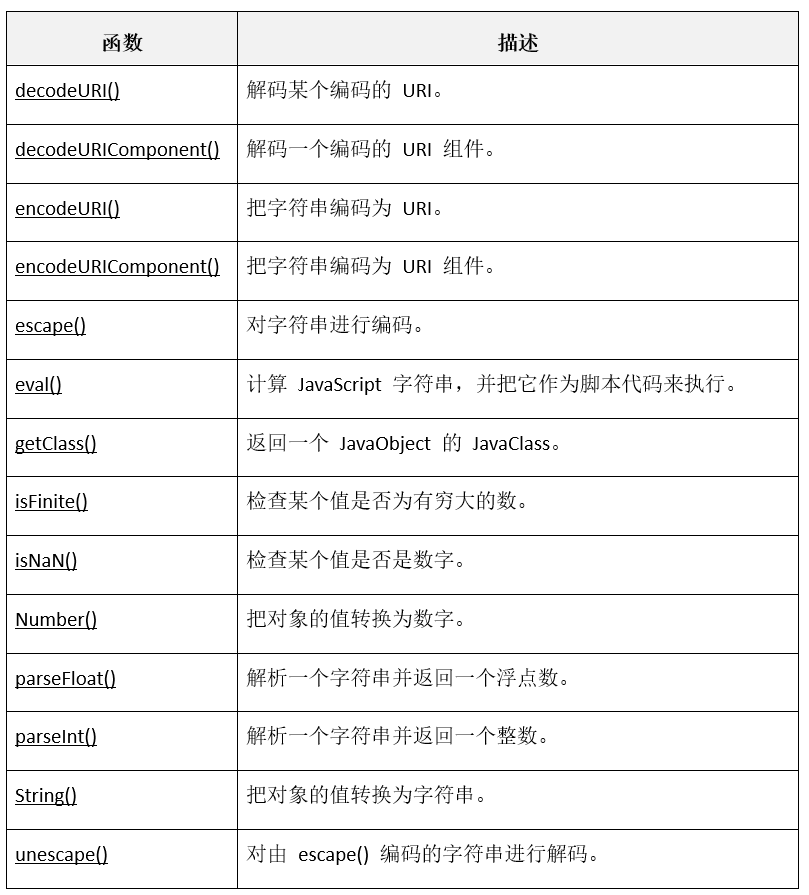JavaScript函数
6119字，阅读需时21分钟

1、定义函数

JavaScript定义函数有两种方式：一种方式是显示定义函数；一种方式是匿名定义函数。

```function functionname(parameters){
函数代码
}```

JS函数不需要声明返回类型，当函数需要返回值时，直接使用return语句返回即可。

```<html>
<title>JavaScript开发案例</title>
<meta http-equiv="Content-Type" content="text/html; charset=utf-8" />
<script type="text/javascript">
num1 = parseInt(document.getElementById("op1").value);
num2 = parseInt(document.getElementById("op2").value);
}
function sum(a,b){
return a+b;
}

</script>
<body>
<h3>JavaScript开发案例</h3>
<hr>
<p> 加数：<input type="text" id="op1" name="op1" maxlength="20" value=""/></p>
<p> 加数：<input type="text" id="op2" name="op2" maxlength="10" value=""/></p>
</form>
</boyd>
<html>```

sum函数是求和函数，计算传入参数a和b的和，并使用return语句返回a和b的和。

parseInt是JS内置函数，该函数将数字型的字符串转换为整数。

```varfun = function(parameters){
函数代码;
}```

```sum = function(a,b){
return a+b;
}```

2、函数的调用

JS有多种方式来调用函数，有直接调用，表达式内调用，在事件响应中调用，通过链接调用。不管哪种方式调用函数，调用时都要写入函数名称，若是匿名函数，要写入变量名称。

```<script type="text/javascript">
num1 = parseInt(document.getElementById("op1").value);
num2 = parseInt(document.getElementById("op2").value);
}
function sum(a,b){
return a+b;
}
</script>```

```function sum(a,b){
return a+b;
}```

sum函数计算a和b两数的和，并使用return语句返回计算结果。调用sum函数就需要在表达式内调用。

```value = sum(10,90)
value = sum(10,90) * 2```

JS代码一般嵌入到HTML网页内，网页的页面加载、用户单击HTML元素、移动光标都会产生事件，若要对产生的事件进行响应或处理，可以调用JS函数来响应这个事件。

```function add(){
num1 = parseInt(document.getElementById("op1").value);
num2 = parseInt(document.getElementById("op2").value);
}```

`<p><input type="button" value="加法" onclick="add()"/></p>`

HTML元素input 的onclick属性用于定义该元素产生单击事件后的动作，onclick属性的值可以是定义的JS函数名称。

JS函数还可以通过超链接调用。在HTML元素<a>标签的href属性的值可以是已定义的JS函数名称，或直接编写JS代码。语法如下：

`JavaScript:函数名称`

```JavaScript:add()

```<html>
<title>JavaScript开发案例</title>
<meta http-equiv="Content-Type" content="text/html; charset=utf-8" />
<script type="text/javascript">
num1 = parseInt(document.getElementById("op1").value);
num2 = parseInt(document.getElementById("op2").value);
}
function sum(a,b){
return a+b;
}
sum(10,100);
</script>
<body>
<h3>JavaScript开发案例</h3>
<hr>
<p> 加数：<input type="text" id="op1" name="op1" /></p>
<p> 加数：<input type="text" id="op2" name="op2" /></p>
</boyd>
<html>```

3、 内置函数

JavaScript内部提供了一些函数，开发者可以直接使用这些函数，提高编程效率和JS程序的稳定性。下表列出了常用的JS内置函数。4、  嵌套函数

JS语言支持函数嵌套，即在函数内部可以嵌套定义子函数。那么嵌套的子函数是否可以在函数外部调用呢？它的作用域又是什么范围呢？

```//定义嵌套函数
function foo() {
m = 3;
function bar() {
n = 4;
}
bar();
}```

```<html>
<title>JavaScript开发案例</title>
<meta http-equiv="Content-Type" content="text/html; charset=utf-8" />
<script type="text/javascript">
function foo() {
m = 3;
function bar() {
n = 4;
}
bar();
}
</script>
<body>
<input type="button" value="调用foo函数" onclick="foo()"/>
<input type="button" value="调用bar函数" onclick="bar()"/>
</boyd>
<html>```

bar函数执行失败，显然在函数体内嵌套的函数是不能被外部调用的，其作用域仅限于函数内部。